n 是代表总人数。

7 5 4
1 3
2 4
3 4
1 4
5 6
1 4
2 3
2 5
6 7


yes
yes
no
no


#include <iostream>
using namespace std;
const int N = 10010;

int f[N];

/*
* 将表示朋友圈的数组初始化，即将所有人的“朋友祖先”都设置为自己的 id ，
* 于是就有了 n 个不同的朋友圈
*/
void init(int n) {
for(int i = 1; i <= n; i++) {
f[i] = i;
}
}

// 得到 id 为 v 的人的“朋友祖先”的 id
int getFriend(int v) {
if(f[v] == v) {
return v;
}
/*
* 如果发现“朋友祖先”不是自己，那么他肯定被合并到别的朋友圈里面去了，
* 那么继续调用这个函数来找这个朋友圈里面的“朋友祖先”，
* 并且在这个过程中将找到的人都设置为同一个“朋友祖先”（因为都在同一个朋友圈里面）
*/
return f[v] = getFriend(f[v]);
}

// 将两个人所在的两个朋友圈合并为一个朋友圈
void merge(int a, int b) {
int t1 = getFriend(a); // 得到左边的人的“朋友祖先”
int t2 = getFriend(b); // 得到右边的人的“朋友祖先”
/* 这里我们制定一个“靠左原则”：一旦发现两个人的“朋友祖先”不一样，
* 那么右边那个人的“朋友祖先”的“朋友祖先”设置为左边的人的“朋友祖先”，
* 当然，也可以制定“靠右原则”
*/
if(t1 != t2) {
f[t2] = t1;
}
}

int main() {
int n, m, k;
cin >> n >> m >> k;
int x, y;
init(n);

for(int i = 0; i < m; i++) {
cin >> x >> y;
merge(x, y);
}
for(int i = 0; i < k; i++) {
cin >> x >> y;
// 如果两个人的“朋友祖先”不相同，证明他们不在同一个朋友圈内，也就不是朋友
if(getFriend(x) != getFriend(y)) {
cout << "no" << endl;
} else {
cout << "yes" << endl;
}
}

return 0;
}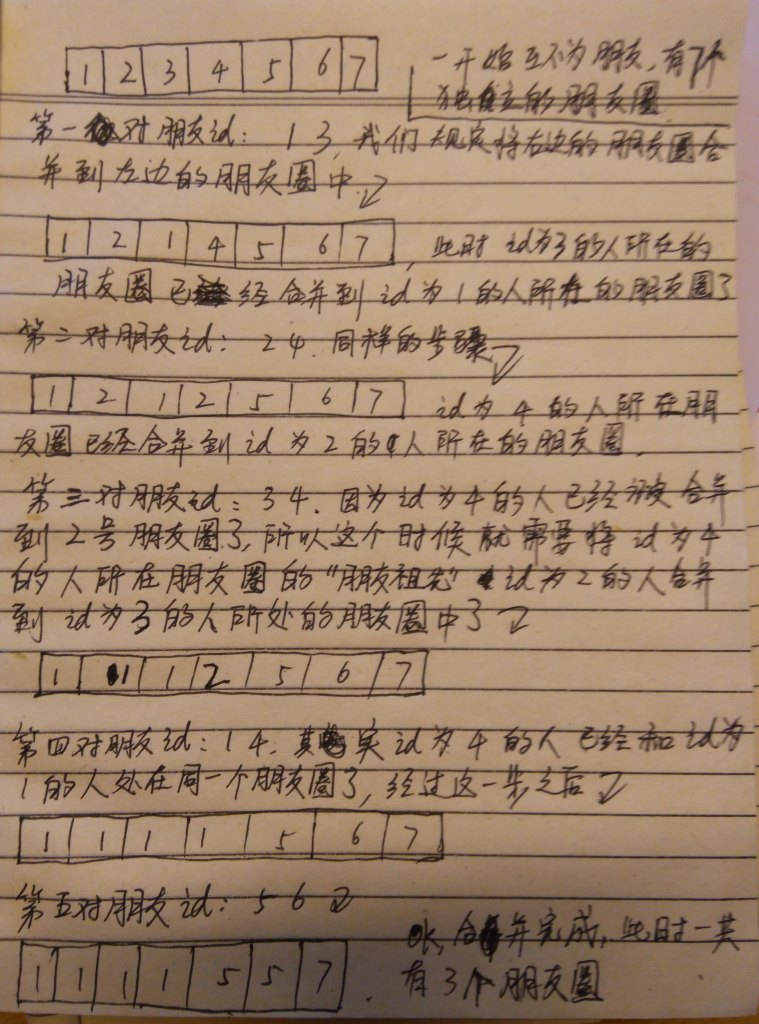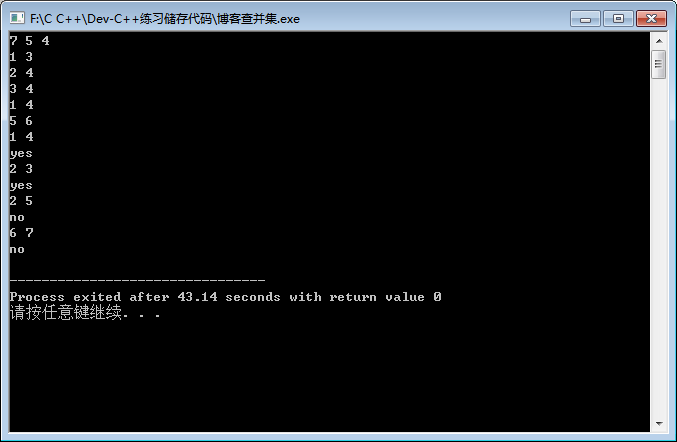#include <iostream>
using namespace std;
const int N = 10010;

int f[N];

/*
* 将表示朋友圈的数组初始化，即将所有人的“朋友祖先”都设置为自己的 id ，
* 于是就有了 n 个不同的朋友圈
*/
void init(int n) {
for(int i = 1; i <= n; i++) {
f[i] = i;
}
}

// 得到 id 为 v 的人的“朋友祖先”的 id
int getFriend(int v) {
if(f[v] == v) {
return v;
}
/*
* 如果发现“朋友祖先”不是自己，那么他肯定被合并到别的朋友圈里面去了，
* 那么继续调用这个函数来找这个朋友圈里面的“朋友祖先”，
* 并且在这个过程中将找到的人都设置为同一个“朋友祖先”（因为都在同一个朋友圈里面）
*/
return f[v] = getFriend(f[v]);
}

// 将两个人所在的两个朋友圈合并为一个朋友圈
void merge(int a, int b) {
int t1 = getFriend(a); // 得到左边的人的“朋友祖先”
int t2 = getFriend(b); // 得到右边的人的“朋友祖先”
/* 这里我们制定一个“靠左原则”：一旦发现两个人的“朋友祖先”不一样，
* 那么右边那个人的“朋友祖先”的“朋友祖先”设置为左边的人的“朋友祖先”，
* 当然，也可以制定“靠右原则”
*/
if(t1 != t2) {
f[t2] = t1;
}
}

int main() {
int n, m, k;
cin >> n >> m >> k;
int x, y;
init(n);

for(int i = 0; i < m; i++) {
cin >> x >> y;
merge(x, y);
}

/*
* 输出合并之后的数组情况
*/
for(int i = 1; i <= n; i++) {
if(i != 1) {
cout << " ";
}
cout << f[i];
}
cout << endl;

for(int i = 0; i < k; i++) {
cin >> x >> y;
// 如果两个人的“朋友祖先”不相同，证明他们不在同一个朋友圈内，也就不是朋友
if(getFriend(x) != getFriend(y)) {
cout << "no" << endl;
} else {
cout << "yes" << endl;
}
}

return 0;

}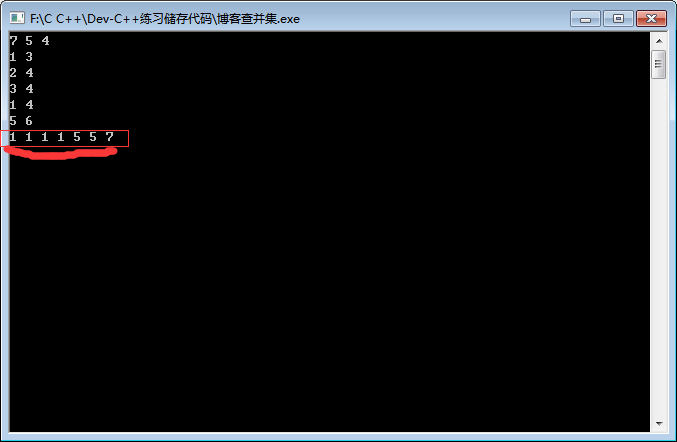Ok，其实上面说的这种算法思想就是查并集，其标准的描述也是通过树和森林来定义的：在一个森林中有很多棵不同的树，我们通过一些信息来将一些不同的分开的树合并成一棵大的树。在这道题目中，一开始森林中有 7 棵不同根节点的树，树的根节点在这道题目中就相当于“朋友祖先”（7 个朋友圈，每个朋友圈中只有一个人，即为他自己，也是每个朋友圈的“朋友祖先”），通过题中所给的信息不断合并朋友圈（合并森林中不同的树），合并结束之后森林中树的棵树或者不同的树的根节点的个数（“朋友祖先”的个数）就是朋友圈的个数。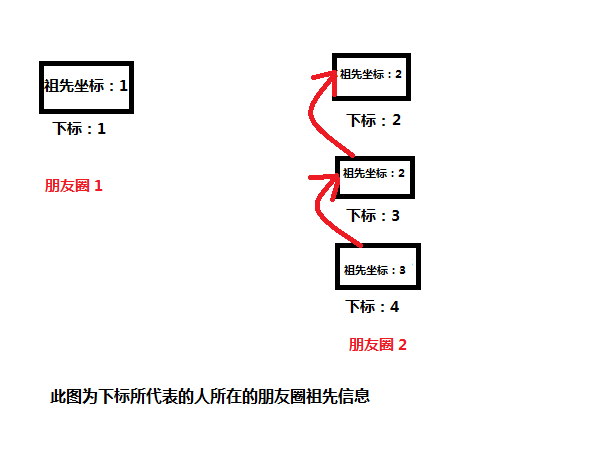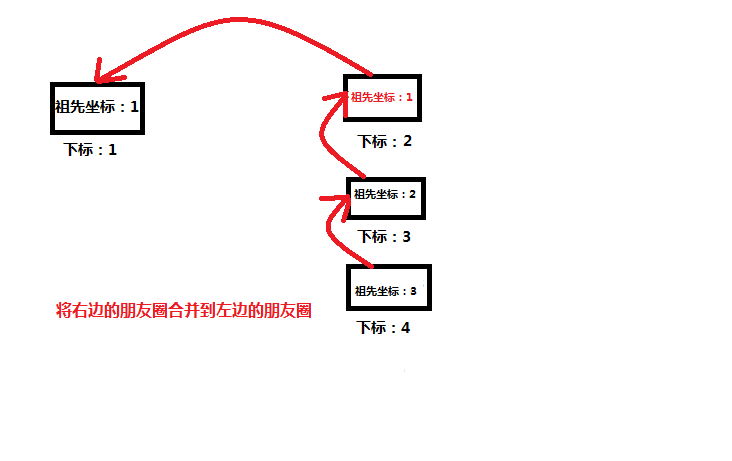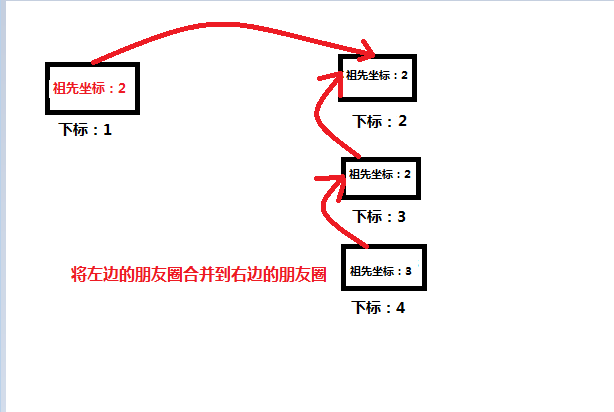int high[N]; // 一个全局数组。保存每个朋友圈的高度，初始时都是 0

// 省略其他代码......

/**
* 将两个人所在的两个朋友圈合并为一个朋友圈
* 这里通过两个朋友圈的高度来决定合并方式
*/
void merge(int a, int b) {
int t1 = getFriend(a); // 得到左边的人的“朋友祖先”
int t2 = getFriend(b); // 得到右边的人的“朋友祖先”
// 两个人的“朋友祖先”不一样，合并两个朋友圈
if(t1 != t2) {
// 如果左边的朋友圈的高度大于右边的朋友圈的高度，
// 那么将右边的朋友圈合并到左边的朋友圈中
if (high[t1] > high[t2]) {
f[t2] = t1;
// 否则就把左边的朋友圈合并到右边的朋友圈中
} else {
f[t1] = t2;
// 如果当前两个朋友圈的高度相等，那么合并之后的朋友圈高度要加一
if (high[t1] == high[t2]) {
high[t2]++;
}
}
}
}

// 省略其他代码......


merge 函数里面有一句注释：// 如果当前两个朋友圈的高度相等，那么合并之后的朋友圈高度要加一 。这句话可能会有点难理解，看一幅图就知道了：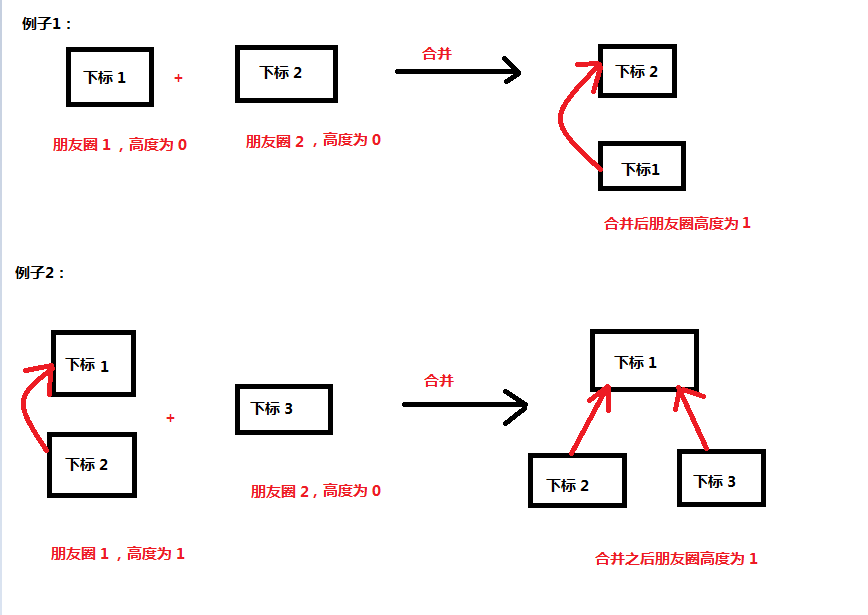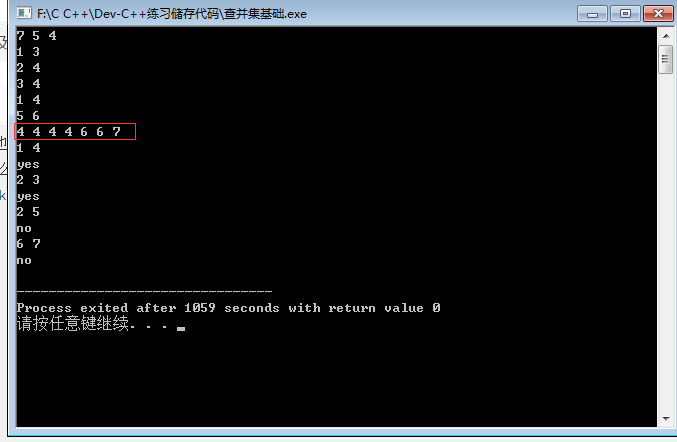#include <iostream>
using namespace std;
const int N = 10010;

int f[N];
int high[N]; // 保存每个朋友圈的高度，初始时都是 0

/*
* 将表示朋友圈的数组初始化，即将所有人的“朋友祖先”都设置为自己的 id ，
* 于是就有了 n 个不同的朋友圈
*/
void init(int n) {
for(int i = 1; i <= n; i++) {
f[i] = i;
}
}

// 得到 id 为 v 的人的“朋友祖先”的 id
int getFriend(int v) {
if(f[v] == v) {
return v;
}
/*
* 如果发现“朋友祖先”不是自己，那么他肯定被合并到别的朋友圈里面去了，
* 那么继续调用这个函数来找这个朋友圈里面的“朋友祖先”，
* 并且在这个过程中将找到的人都设置为同一个“朋友祖先”（因为都在同一个朋友圈里面）
*/
return f[v] = getFriend(f[v]);
}

/**
* 将两个人所在的两个朋友圈合并为一个朋友圈
* 这里通过两个朋友圈的高度来决定合并方式
*/
void merge(int a, int b) {
int t1 = getFriend(a); // 得到左边的人的“朋友祖先”
int t2 = getFriend(b); // 得到右边的人的“朋友祖先”
// 两个人的“朋友祖先”不一样，合并两个朋友圈
if(t1 != t2) {
// 如果左边的朋友圈的高度大于右边的朋友圈的高度，
// 那么将右边的朋友圈合并到左边的朋友圈中
if (high[t1] > high[t2]) {
f[t2] = t1;
// 否则就把左边的朋友圈合并到右边的朋友圈中
} else {
f[t1] = t2;
// 如果当前两个朋友圈的高度相等，那么合并之后的朋友圈高度要加一，
if (high[t1] == high[t2]) {
high[t2]++;
}
}
}
}

int main() {
int n, m, k;
cin >> n >> m >> k;
int x, y;
init(n);

for(int i = 0; i < m; i++) {
cin >> x >> y;
merge(x, y);
}

/*
* 输出合并之后的数组情况
*/
for(int i = 1; i <= n; i++) {
if(i != 1) {
cout << " ";
}
cout << f[i];
}
cout << endl;

for(int i = 0; i < k; i++) {
cin >> x >> y;
// 如果两个人的“朋友祖先”不相同，证明他们不在同一个朋友圈内，也就不是朋友
if(getFriend(x) != getFriend(y)) {
cout << "no" << endl;
} else {
cout << "yes" << endl;
}
}

return 0;

}


## 模板题

10 9
1 2
1 3
1 4
1 5
1 6
1 7
1 8
1 9
1 10
10 4
2 3
4 5
4 8
5 8
0 0


Case 1: 1
Case 2: 7


AC代码：

#include<iostream>
using namespace std;
const int N=50010;
int f[N];
int res=0;
void init(int n){
for(int i=1;i<=n;i++){
f[i]=i;
}
}
int getfriend(int v){
if(f[v]==v) return v;
else return f[v]=getfriend(f[v]);
}
void merge(int a,int b){

int x=getfriend(a);
int y=getfriend(b);
if(x!=y){
f[y]=x;
res++;
}
}
int main()
{
int n,m,k=0,x,y;
while(1){
res=0;
k++;
cin>>n>>m;
if(n==0&&m==0) break;
init(n);
for(int i=1;i<=m;i++){
cin>>x>>y;
merge(x,y);
}
cout<<"Case "<<k<<": ";
cout<<n-res<<endl;
}

return 0;
}


••